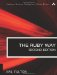# Section 5.20. Determining the Architecture s Byte Order

### 5.19. Finding Cube Roots, Fourth Roots, and so on

Ruby has a built-in square root function (Math.sqrt) because that function is so commonly used. But what if you need higher level roots? If you remember your math, this is easy.

One way is to use logarithms. Recall that e to the x is the inverse of the natural log of x, and that when we multiply numbers, that is equivalent to adding their logarithms.

`x = 531441 cuberoot = Math.exp(Math.log(x)/3.0)     # 81.0 fourthroot = Math.exp(Math.log(x)/4.0)   # 27.0`

However, it is just as easy and perhaps clearer simply to use fractions with an exponentiation operator (which can take any integer or floating point value).

`include Math y = 4096 cuberoot = y**(1.0/3.0)      # 16.0 fourthroot = y**(1.0/4.0)    # 8.0 fourthroot = sqrt(sqrt(y))   # 8.0 (same thing) twelfthroot = y**(1.0/12.0)  # 2.0`

Note that in all these examples, we have used floating point numbers when dividing (to avoid truncation to an integer).The Ruby Way, Second Edition: Solutions and Techniques in Ruby Programming (2nd Edition)
ISBN: 0672328844
EAN: 2147483647
Year: 2004
Pages: 269
Authors: Hal Fulton

Similar book on Amazon